## Miller Effect in Amplifier Frequency Response:

According to this effect, when viewed from the input base terminal of the CE-connected transistor the capacitance Ccb appears as (1 – Av)Ccb i.e., it is amplified by a factor of (1 – Av). In fact Miller effect takes into account the feedback from the collector to base and vice versa due to Ccb.

When input signal of voltage Vin is applied to the base of the transistor, the change in collector voltage,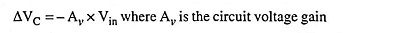The negative sign is due to phase shift inherent in a CE amplifier. As seen, collector voltage VC is reduced by (AvVin) when base voltage is increased by Vin. Thus total reduction in collector-base voltage is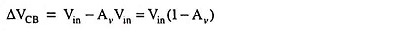This also represents the change of voltage across Ccb, the capacitance between collector and base. Now charge supplied to the input of the circuit,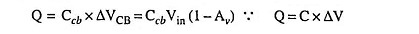Thus, “looking into” the base, it is seen that the collector-base capacitance appears to be (1 – Av)Ccb; i.e., the capacitance is amplified by a factor of (1 – Av). This amplification is known as the Miller effect.

The total input capacitance Cin to the transistor is (1 – Av)Ccb in parallel with Cbe. Thus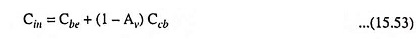At high frequencies, the value of Cin reduces the input impedance Zin of the circuit and affects the frequency response.

Noteworthy point is that Miller effect occurs only with a circuit in which there is a phase reversal such as in CE configuration. It does not occur in CB and CC configurations.

In the same way, the device inter-terminal capacitances (Cgs, Cgd and Cds) are important quantities in determination of the performance of a FET circuit, and these are used in the same way as the BJT capacitances. The input capacitance is amplified by the Miller effect in the case of a common source circuit (an inverting amplifier). Equation (15.53) may be written in the FET circuit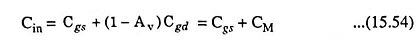where CM is the Miller capacitance and is equal to (1 – Av)Cgd.

Scroll to Top